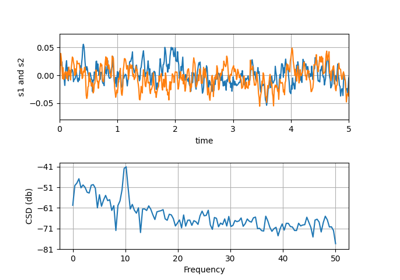# matplotlib.axes.Axes.csd¶

Axes.csd(self, x, y, NFFT=None, Fs=None, Fc=None, detrend=None, window=None, noverlap=None, pad_to=None, sides=None, scale_by_freq=None, return_line=None, *, data=None, **kwargs)[source]

Plot the cross-spectral density.

The cross spectral density $$P_{xy}$$ by Welch's average periodogram method. The vectors x and y are divided into NFFT length segments. Each segment is detrended by function detrend and windowed by function window. noverlap gives the length of the overlap between segments. The product of the direct FFTs of x and y are averaged over each segment to compute $$P_{xy}$$, with a scaling to correct for power loss due to windowing.

If len(x) < NFFT or len(y) < NFFT, they will be zero padded to NFFT.

Parameters:
x, y1-D arrays or sequences

Arrays or sequences containing the data.

Fsscalar

The sampling frequency (samples per time unit). It is used to calculate the Fourier frequencies, freqs, in cycles per time unit. The default value is 2.

windowcallable or ndarray

A function or a vector of length NFFT. To create window vectors see window_hanning, window_none, numpy.blackman, numpy.hamming, numpy.bartlett, scipy.signal, scipy.signal.get_window, etc. The default is window_hanning. If a function is passed as the argument, it must take a data segment as an argument and return the windowed version of the segment.

sides{'default', 'onesided', 'twosided'}

Specifies which sides of the spectrum to return. Default gives the default behavior, which returns one-sided for real data and both for complex data. 'onesided' forces the return of a one-sided spectrum, while 'twosided' forces two-sided.

The number of points to which the data segment is padded when performing the FFT. This can be different from NFFT, which specifies the number of data points used. While not increasing the actual resolution of the spectrum (the minimum distance between resolvable peaks), this can give more points in the plot, allowing for more detail. This corresponds to the n parameter in the call to fft(). The default is None, which sets pad_to equal to NFFT

NFFTint

The number of data points used in each block for the FFT. A power 2 is most efficient. The default value is 256. This should NOT be used to get zero padding, or the scaling of the result will be incorrect. Use pad_to for this instead.

detrend{'none', 'mean', 'linear'} or callable, default 'none'

The function applied to each segment before fft-ing, designed to remove the mean or linear trend. Unlike in MATLAB, where the detrend parameter is a vector, in Matplotlib is it a function. The mlab module defines detrend_none, detrend_mean, and detrend_linear, but you can use a custom function as well. You can also use a string to choose one of the functions: 'none' calls detrend_none. 'mean' calls detrend_mean. 'linear' calls detrend_linear.

scale_by_freqbool, optional

Specifies whether the resulting density values should be scaled by the scaling frequency, which gives density in units of Hz^-1. This allows for integration over the returned frequency values. The default is True for MATLAB compatibility.

noverlapint

The number of points of overlap between segments. The default value is 0 (no overlap).

Fcint

The center frequency of x (defaults to 0), which offsets the x extents of the plot to reflect the frequency range used when a signal is acquired and then filtered and downsampled to baseband.

return_linebool

Whether to include the line object plotted in the returned values. Default is False.

Returns:
Pxy1-D array

The values for the cross spectrum P_{xy} before scaling (complex valued).

freqs1-D array

The frequencies corresponding to the elements in Pxy.

lineLine2D

The line created by this function. Only returned if return_line is True.

Other Parameters:
**kwargs

Keyword arguments control the Line2D properties:

Property Description
agg_filter a filter function, which takes a (m, n, 3) float array and a dpi value, and returns a (m, n, 3) array
alpha float or None
animated bool
antialiased or aa bool
clip_box Bbox
clip_on bool
clip_path Patch or (Path, Transform) or None
color or c color
contains callable
dash_capstyle {'butt', 'round', 'projecting'}
dash_joinstyle {'miter', 'round', 'bevel'}
dashes sequence of floats (on/off ink in points) or (None, None)
data (2, N) array or two 1D arrays
drawstyle or ds {'default', 'steps', 'steps-pre', 'steps-mid', 'steps-post'}, default: 'default'
figure Figure
fillstyle {'full', 'left', 'right', 'bottom', 'top', 'none'}
gid str
in_layout bool
label object
linestyle or ls {'-', '--', '-.', ':', '', (offset, on-off-seq), ...}
linewidth or lw float
marker marker style
markeredgecolor or mec color
markeredgewidth or mew float
markerfacecolor or mfc color
markerfacecoloralt or mfcalt color
markersize or ms float
markevery None or int or (int, int) or slice or List[int] or float or (float, float)
path_effects AbstractPathEffect
picker float or callable[[Artist, Event], Tuple[bool, dict]]
pickradius float
rasterized bool or None
sketch_params (scale: float, length: float, randomness: float)
snap bool or None
solid_capstyle {'butt', 'round', 'projecting'}
solid_joinstyle {'miter', 'round', 'bevel'}
transform matplotlib.transforms.Transform
url str
visible bool
xdata 1D array
ydata 1D array
zorder float

psd()
psd() is the equivalent to setting y=x.

Notes

For plotting, the power is plotted as $$10 \log_{10}(P_{xy})$$ for decibels, though P_{xy} itself is returned.

References

Bendat & Piersol -- Random Data: Analysis and Measurement Procedures, John Wiley & Sons (1986)

Note

In addition to the above described arguments, this function can take a data keyword argument. If such a data argument is given, the following arguments are replaced by data[<arg>]:

• All arguments with the following names: 'x', 'y'.

Objects passed as data must support item access (data[<arg>]) and membership test (<arg> in data).

## Examples using matplotlib.axes.Axes.csd¶